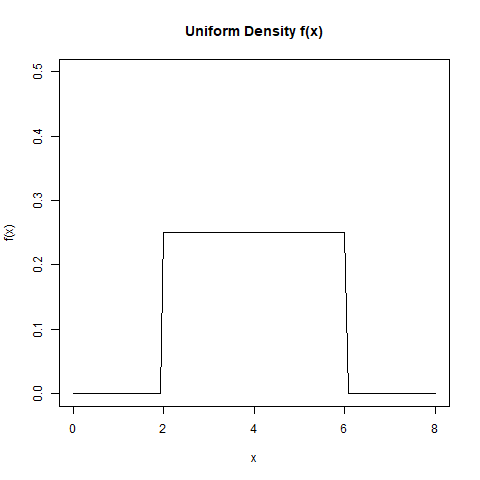Related Articles
Compute Density of the Distribution Function in R Programming – dunif() Function
• Last Updated : 30 Jun, 2020

`dunif()` function in R Language is used to provide the density of the distribution function.

Syntax:
dunif(x, min = 0, max = 1, log = FALSE)

Parameters:
x: represents vector
min, max: represents lower and upper limits of the distribution
log: represents logical value for probabilities

Example 1:

 `# Create vector of random deviation``u <- ``runif``(20)`` ` `dunif``(u) == u`` ` `print``(``dunif``(u))`

Output:

```
 FALSE FALSE FALSE FALSE FALSE FALSE FALSE FALSE FALSE FALSE FALSE FALSE
 FALSE FALSE FALSE FALSE FALSE FALSE FALSE FALSE
 1 1 1 1 1 1 1 1 1 1 1 1 1 1 1 1 1 1 1 1
```

Example 2:

 `# Output to be present as PNG file``png``(file = ``"dunifGFG.png"``)`` ` `# Plot density``curve``(``dunif``(x, min = 2, max = 6), 0, 8, ylim = ``c``(0, 0.5),``      ``ylab = ``"f(x)"``, main = ``"Uniform Density f(x)"``)`` ` `# Saving the file``dev.off``()`

Output:My Personal Notes arrow_drop_up